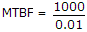# Electronics and Communication Engineering - Microprocessors

### Exercise :: Microprocessors - Section 3

41.

Which of the following is operating system?

 A. MS WORD B. Ventura C. Java D. Windows

Explanation:

Windows is an operating system.

42.

A modem is connected to PC at

 A. serial port B. parallel port C. SCSI port D. input port

Explanation:

Modem is connected at serial port.

43.

Assertion (A): The data bus and address bus of 8085 are multiplexed.

Reason (R): Multiplexing reduces the number of pins.

 A. Both A and R are correct and R is correct explanation of A B. Both A and R are correct but R is not correct explanation of A C. A is correct R is wrong D. A is wrong R is correct

Explanation:

In 8085 signal lines AD7 - AD0 are used for address bits in some T states and for data in some other T states.

44.

Consider the following statements about 'Functions' in C

1. A function can be called any number of times.
2. A function gets called when the function name is followed by a semicolon.
3. The order in which functions are defined in a program and the order in which they are called must be the same.
Which of the above statements are correct?

 A. All B. 1 and 2 only C. 1 and 3 only D. 2 and 3 only

Explanation:

The two orders need not be the same in C.

45.

A failure rate of 0.01 percent in 1000 hours in a memory corresponds to an MTBF of

 A. 1000 hours B. 100000 hours C. 10 hours D. 100 hours.